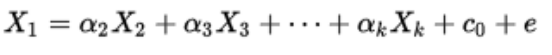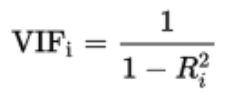# What is VIF? How do you calculate it?

VIF stands for Variance inflation factor, which measures how much variance of an estimated regression coefficient is increased due to the presence of collinearity between the variables. It also determines how much multicollinearity exists in a particular regression model.

### Calculation of VIF:

Firstly, it applies the ordinary least square method of regression that has Xi as a function of all the other explanatory or independent variables and then calculates VIF using the given below mathematical formula: